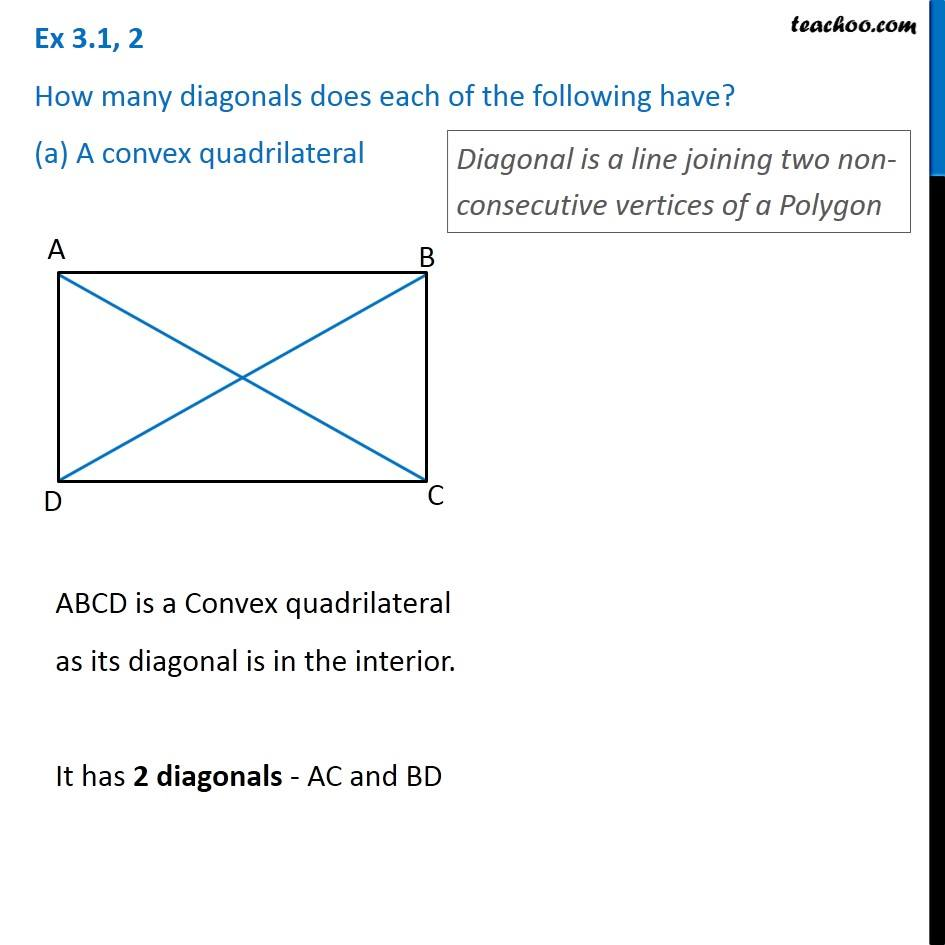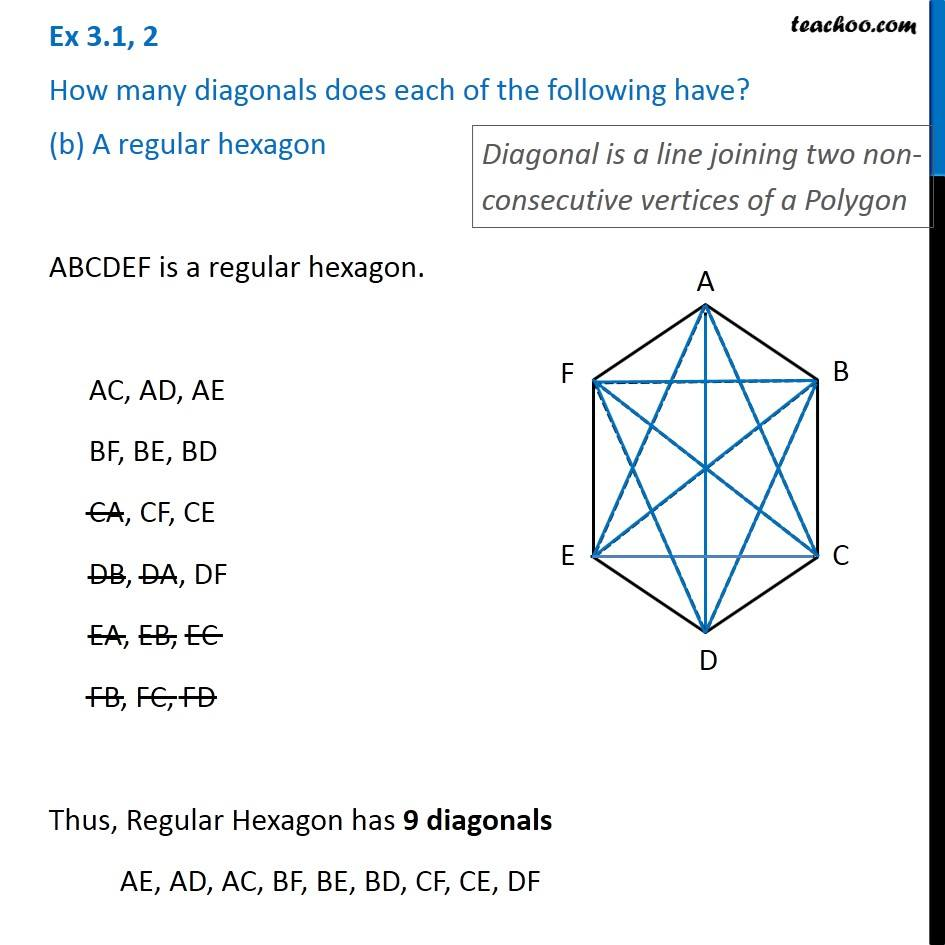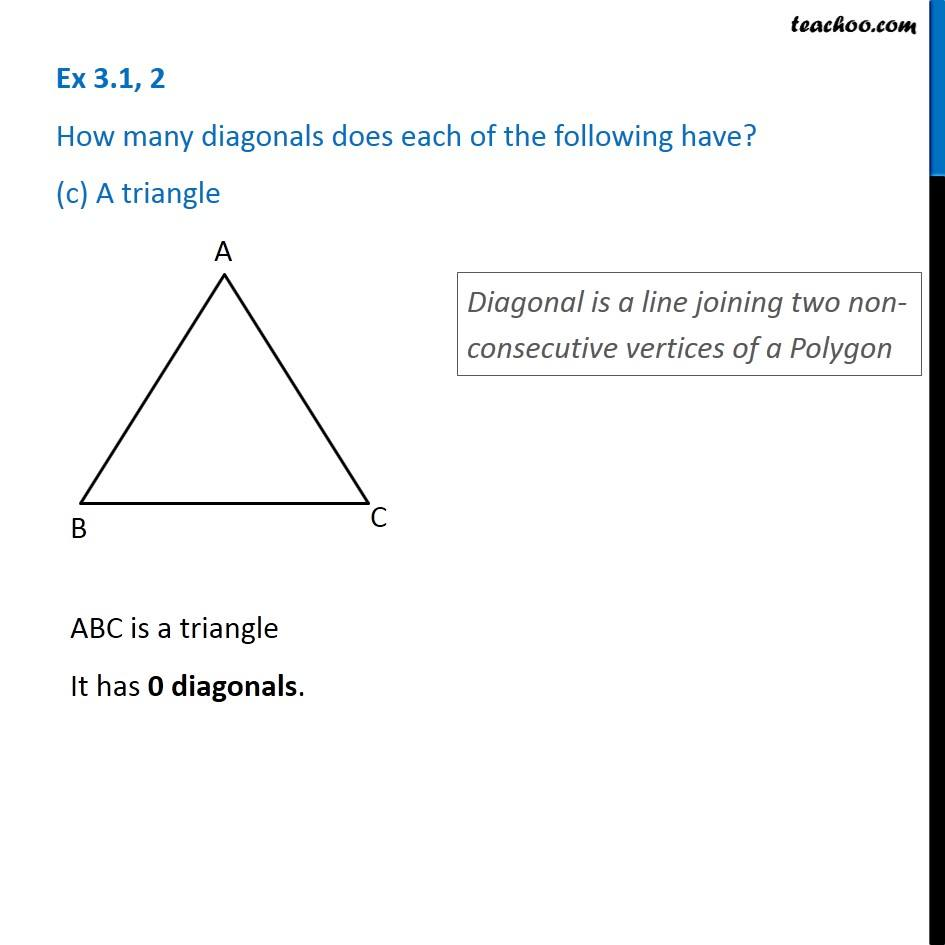Subscribe to our Youtube Channel - https://you.tube/teachoo

1. Chapter 3 Class 8 Understanding Quadrilaterals
2. Serial order wise
3. Ex 3.1

Transcript

Ex 3.1, 2 How many diagonals does each of the following have? (a) A convex quadrilateral Diagonal is a line joining two non-consecutive vertices of a Polygon ABCD is a Convex quadrilateral as its diagonal is in the interior. It has 2 diagonals - AC and BD Ex 3.1, 2 How many diagonals does each of the following have? (b) A regular hexagon Diagonal is a line joining two non-consecutive vertices of a Polygon ABCDEF is a regular hexagon. AC, AD, AE BF, BE, BD CA, CF, CE DB, DA, DF EA, EB, EC FB, FC, FD Thus, Regular Hexagon has 9 diagonals AE, AD, AC, BF, BE, BD, CF, CE, DF Ex 3.1, 2 How many diagonals does each of the following have? (c) A triangleDiagonal is a line joining two non-consecutive vertices of a Polygon ABC is a triangle It has 0 diagonals.

Ex 3.1